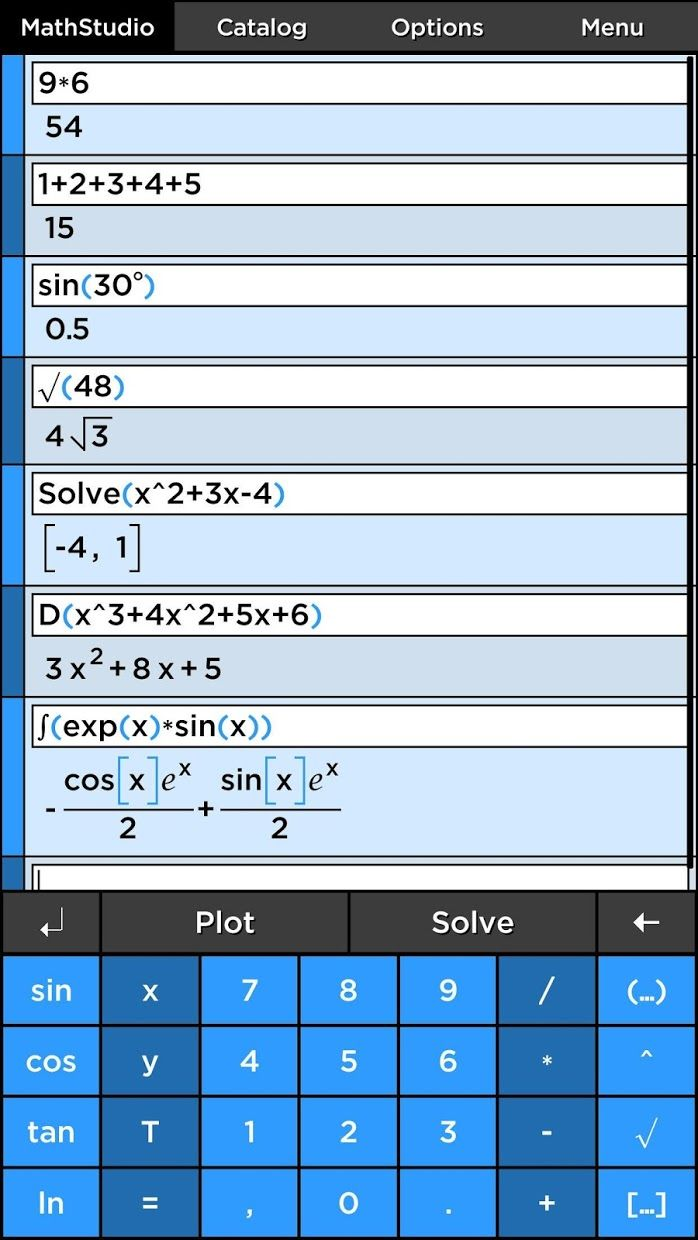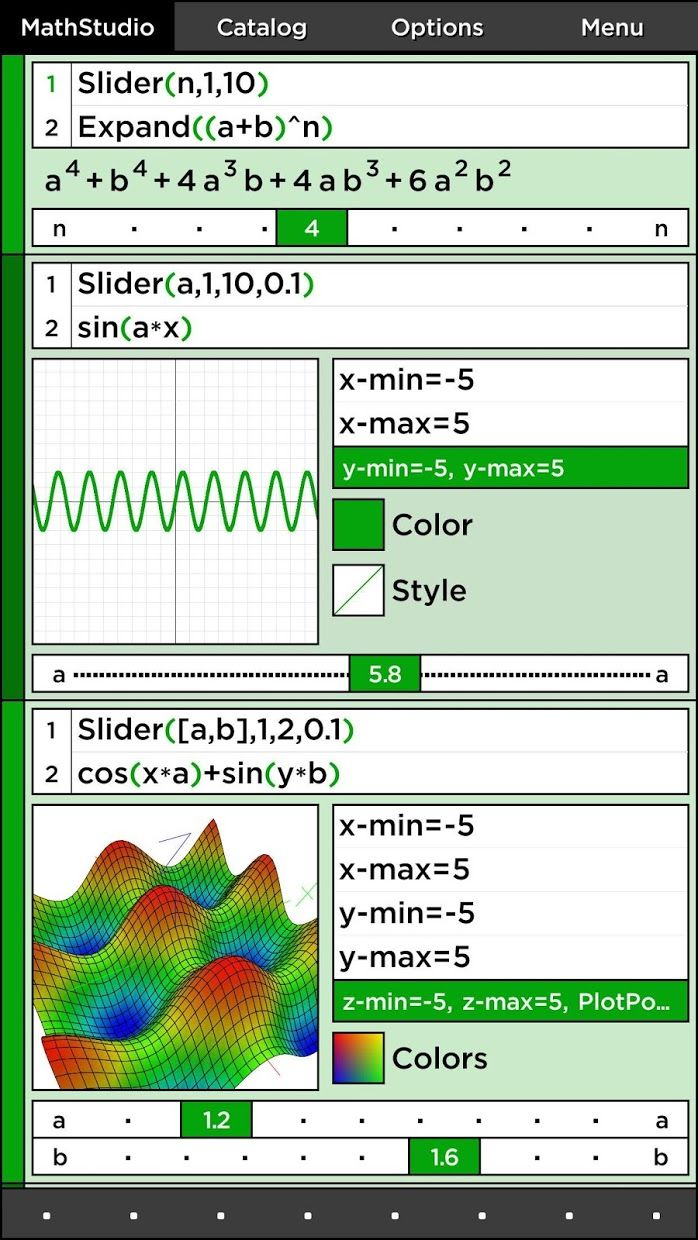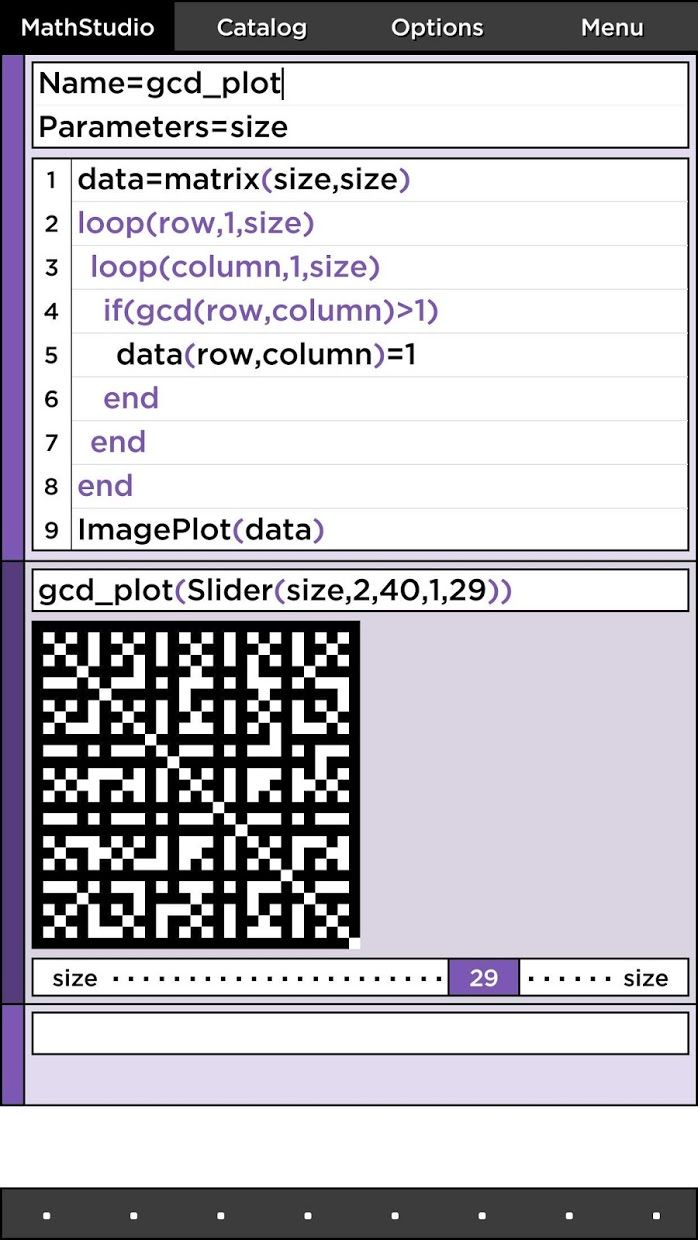# MathStudio Express

4.6

10,000+人安装

### 截图

•••••### 详情

```Whether you need a simple calculator to do your finances or a replacement for your TI graphing calculator, MathStudio Express is a full featured scientific calculator with hundreds of mathematical functions that cover Basic Math, Pre-Algebra, Algebra, Trigonometry, Precalculus, Calculus, Statistics, Finite Math, Linear Algebra and Statistics.

"MathStudio is a natural for students and for engineers who occasionally need a comprehensive math program but don't need the full horsepower and daunting complexity of Mathematica or Maple. It's a huge leap from the usual calculator program for tablets and smartphones." - IEEE Spectrum Review by Kenneth R. Foster
http://spectrum.ieee.org/computing/software/review-mathstudio

MathStudio Express is an indispensable tool for students, teachers and engineers. It provides features comparable to TI calculators but with a modern and intuitive interface.

Graph equations with speed and ease you never thought was possible! Move, rotate and pinch function, parametric, polar, implicit, contour, spherical, cylindrical, vector fields, fractals and image plots with amazing speed and accuracy. Use the T variable to create animated plots that graph in space and time!

Plot lists of numerical data in scatter, histograms, box and probability plots. MathStudio even includes an advanced regression analysis package, choose from several regression functions or create your own.

MathStudio Express is powered by that fastest proprietary computer algebra system available on Android that beautifully typesets your answers. Got a tough algebra equation to solve or polynomial to factor? Solve quadratic equations, systems of equations, limits, derivates, integrals, differential equations and much more. Create scripts in MathStudio's powerful programming language that supports variables, loops and recursion.

Are you a user from Mathematica or MATLAB? MathStudio Express offers much of the same functionality. For more help getting starting using MathStudio Express visit our forums.
http://discuss.mathstud.io

“MathStudio对于学生和偶尔需要全面数学课程但不需要Mathematica或Maple的全部功能和令人生畏的复杂性的工程师来说是很自然的。它通常用于平板电脑和智能手机的计算器程序。” - Kenneth R. Foster的IEEE Spectrum Review
http://spectrum.ieee.org/computing/software/review-mathstudio

MathStudio Express是学生，教师和工程师不可或缺的工具。它提供了与TI计算器相媲美的功能，但具有现代直观的界面。

MathStudio Express由Android上最快的专有计算机代数系统提供支持，可以很好地排版您的答案。有一个强大的代数方程求解或多项式因子？求解二次方程，方程组，极限，导数，积分，微分方程等等。使用MathStudio强大的编程语言创建脚本，支持变量，循环和递归。

http://discuss.mathstud.io
```

### 新版本特征

6.0.5

2016年7月1日

### 用户评价国内唯一海外游戏及应用下载平台自动配置谷歌套件全球游戏及应用便捷下载全球网络免费加速